/

### Deriving the principal components of two uncorrelated return series

Suppose the returns on two uncorrelated series are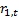and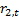. It is assumed that we want an analytical solution rather than a numerical solution (a numerical solution can be found using Nematrian web services functions that target principal components, i.e. MnPrincipalComponents, MnPrincipalComponentsSizes and MnPrincipalComponentsWeights).

For a two series problem, if the covariance matrix is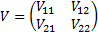then the principal components are associated with the eigenvectors and eigenvalues of the covariance matrix, i.e. with values of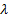that satisfy, for some vector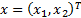the equation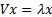. Thetherefore satisfy the following equations: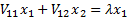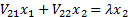This means that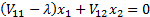and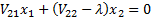, i.e. (since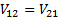for a covariance matrix):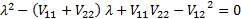In this instance, the two series are uncorrelated and therefore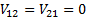. The quadratic then becomes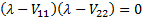, i.e.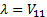or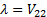. The (population) covariance matrix is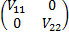, which thus has two eigenvalues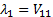and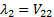and associated eigenvectors which are of the form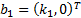and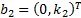respectively for arbitrary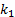and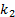. The first principal component is associated with whichever of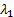and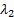is the larger, and the second principal component with the other one.

If we want principal components that are orthonormal return series and portfolio exposures that correspond to these principal components then the portfolio exposures must have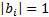for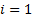and 2, which means in this instance that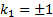and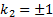. If we choose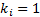then the resulting return series are merely de-meaned versions of the original series, i.e. are are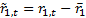and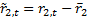or vice-versa depending on whether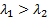or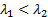.